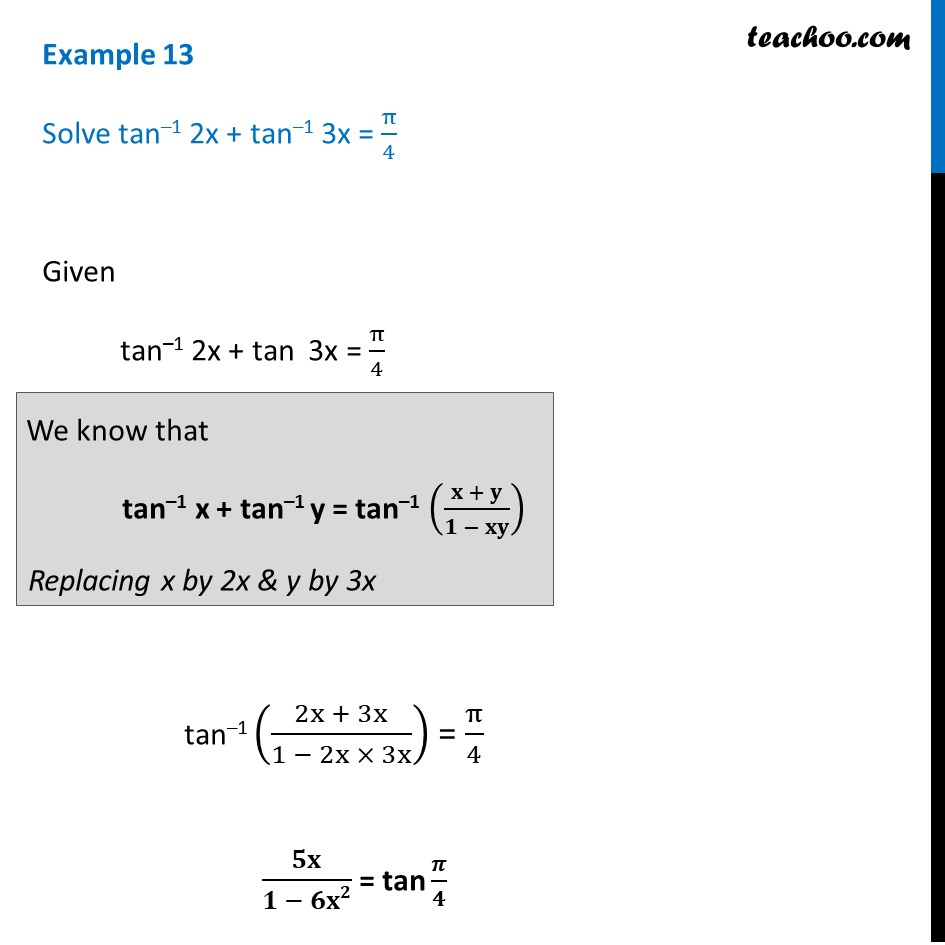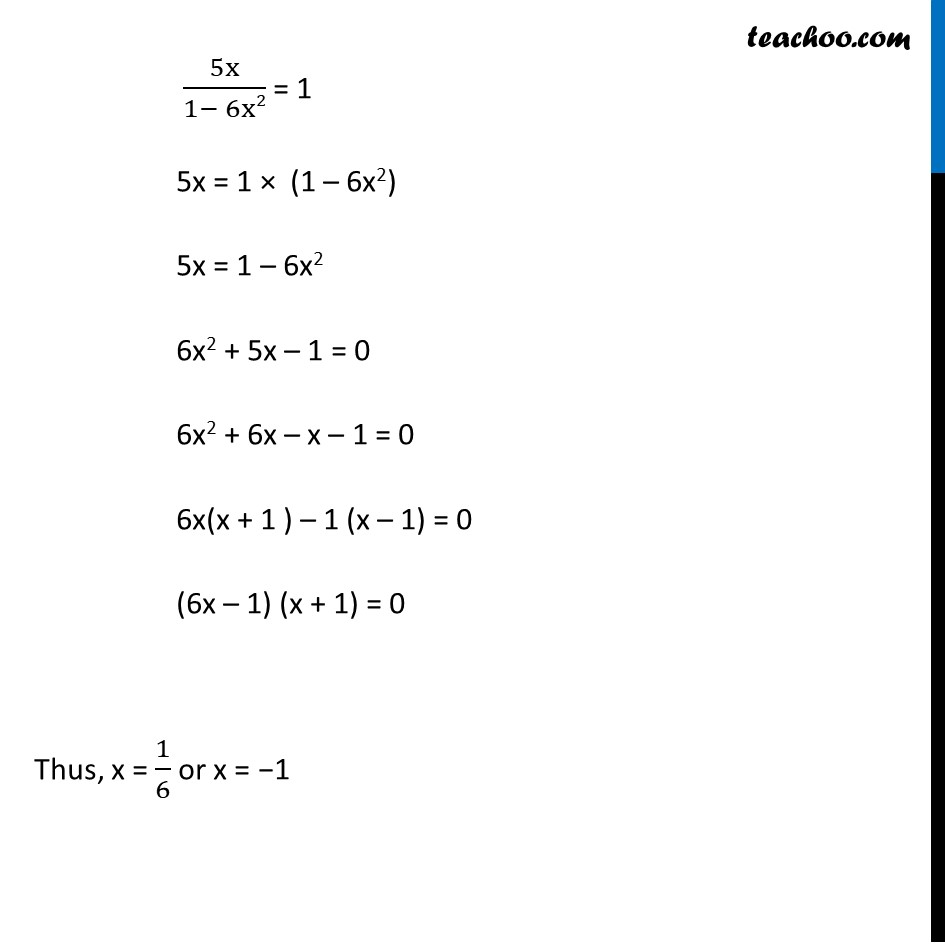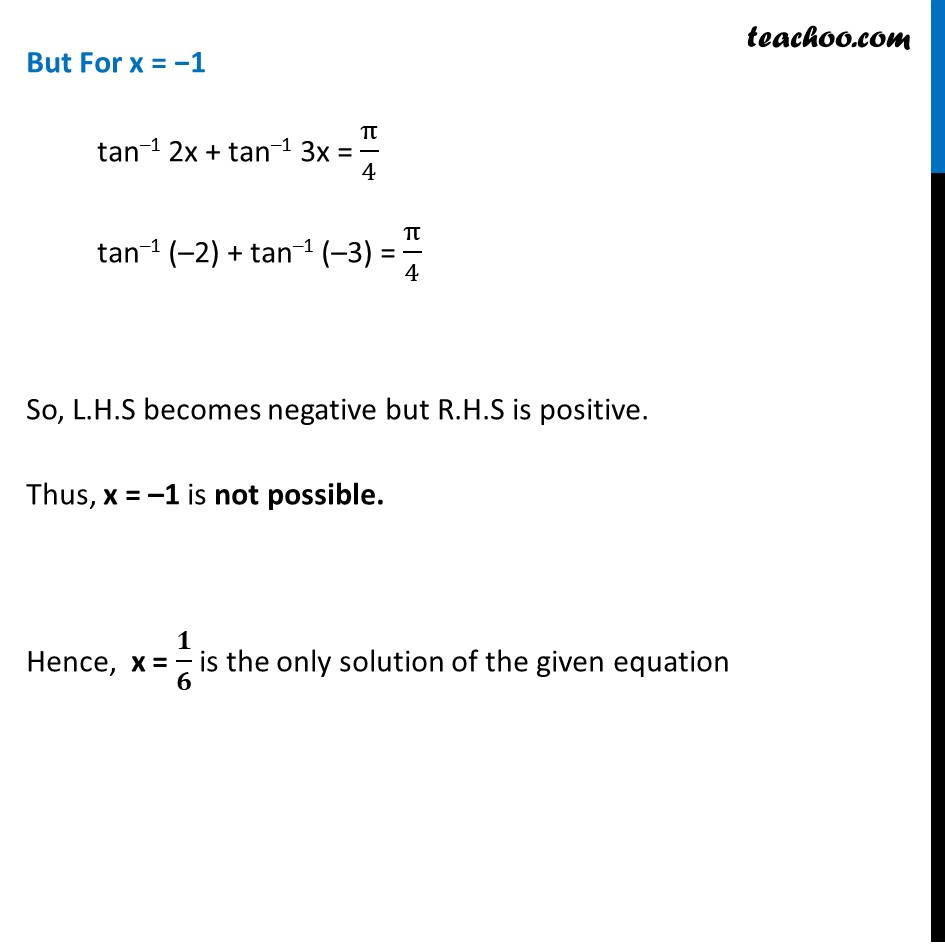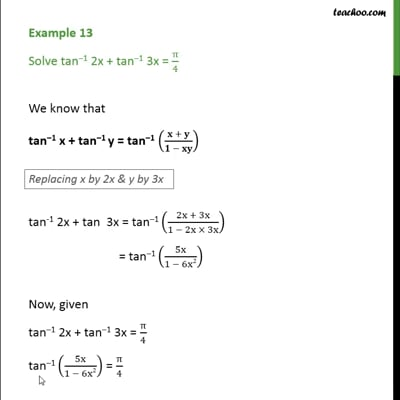Examples

Chapter 2 Class 12 Inverse Trigonometric Functions
Serial order wiseThis video is only available for Teachoo black users

Introducing your new favourite teacher - Teachoo Black, at only ₹83 per month

### Transcript

Example 13 Solve tan–1 2x + tan–1 3x = π/4 Given tan–1 2x + tan 3x = π/4 tan–1 ((2x + 3x)/(1 − 2x × 3x)) = π/4 𝟓𝐱/(𝟏 − 𝟔𝐱𝟐) = tan 𝝅/𝟒 We know that tan–1 x + tan–1 y = tan–1 ((𝐱 + 𝐲)/(𝟏 − 𝐱𝐲)) Replacing x by 2x & y by 3x 5x/(1− 6x2) = 1 5x = 1 × (1 – 6x2) 5x = 1 – 6x2 6x2 + 5x – 1 = 0 6x2 + 6x – x – 1 = 0 6x(x + 1 ) – 1 (x – 1) = 0 (6x – 1) (x + 1) = 0 Thus, x = 1/6 or x = −1 But For x = −1 tan–1 2x + tan–1 3x = π/4 tan–1 (–2) + tan–1 (–3) = π/4 So, L.H.S becomes negative but R.H.S is positive. Thus, x = –1 is not possible. Hence, x = 𝟏/𝟔 is the only solution of the given equation